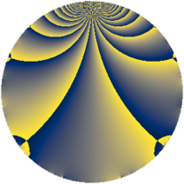Properties

 Label 825.2.fLevel $825$ Weight $2$ Character orbit 825.f Rep. character $\chi_{825}(626,\cdot)$ Character field $\Q$ Dimension $70$ Newform subspaces $7$ Sturm bound $240$ Trace bound $6$

Related objects

Defining parameters

 Level: $$N$$ $$=$$ $$825 = 3 \cdot 5^{2} \cdot 11$$ Weight: $$k$$ $$=$$ $$2$$ Character orbit: $$[\chi]$$ $$=$$ 825.f (of order $$2$$ and degree $$1$$) Character conductor: $$\operatorname{cond}(\chi)$$ $$=$$ $$33$$ Character field: $$\Q$$ Newform subspaces: $$7$$ Sturm bound: $$240$$ Trace bound: $$6$$ Distinguishing $$T_p$$: $$2$$, $$23$$, $$37$$

Dimensions

The following table gives the dimensions of various subspaces of $$M_{2}(825, [\chi])$$.

Total New Old
Modular forms 132 82 50
Cusp forms 108 70 38
Eisenstein series 24 12 12

Trace form

 $$70 q + 3 q^{3} + 68 q^{4} - 7 q^{9} + O(q^{10})$$ $$70 q + 3 q^{3} + 68 q^{4} - 7 q^{9} + 14 q^{12} + 56 q^{16} + 8 q^{22} - 12 q^{27} - 34 q^{31} + 7 q^{33} + 8 q^{34} - 58 q^{36} - 2 q^{37} + 8 q^{42} + 48 q^{48} - 46 q^{49} - 8 q^{58} + 40 q^{64} - 84 q^{66} - 62 q^{67} + 89 q^{69} - 88 q^{78} - 63 q^{81} + 56 q^{82} - 16 q^{88} + 16 q^{91} + 51 q^{93} + 14 q^{97} + 3 q^{99} + O(q^{100})$$

Decomposition of $$S_{2}^{\mathrm{new}}(825, [\chi])$$ into newform subspaces

Label Dim. $$A$$ Field CM Traces $q$-expansion
$a_{2}$ $a_{3}$ $a_{5}$ $a_{7}$
825.2.f.a $2$ $6.588$ $$\Q(\sqrt{-11})$$ $$\Q(\sqrt{-11})$$ $$0$$ $$-1$$ $$0$$ $$0$$ $$q-\beta q^{3}-2q^{4}+(-3+\beta )q^{9}+(-1+\cdots)q^{11}+\cdots$$
825.2.f.b $4$ $6.588$ $$\Q(i, \sqrt{11})$$ $$\Q(\sqrt{-11})$$ $$0$$ $$0$$ $$0$$ $$0$$ $$q-\beta _{1}q^{3}-2q^{4}+(3+\beta _{2})q^{9}+(1+2\beta _{2}+\cdots)q^{11}+\cdots$$
825.2.f.c $8$ $6.588$ 8.0.619810816.2 None $$0$$ $$2$$ $$0$$ $$0$$ $$q+(\beta _{3}+\beta _{4})q^{2}+(-\beta _{3}-\beta _{5})q^{3}+(1+\cdots)q^{4}+\cdots$$
825.2.f.d $8$ $6.588$ 8.0.619810816.2 None $$0$$ $$2$$ $$0$$ $$0$$ $$q+(-\beta _{3}-\beta _{4})q^{2}-\beta _{4}q^{3}+(1-\beta _{4}+\cdots)q^{4}+\cdots$$
825.2.f.e $16$ $6.588$ $$\mathbb{Q}[x]/(x^{16} - \cdots)$$ None $$0$$ $$-2$$ $$0$$ $$0$$ $$q+\beta _{3}q^{2}+\beta _{4}q^{3}+(1+\beta _{1})q^{4}+\beta _{5}q^{6}+\cdots$$
825.2.f.f $16$ $6.588$ $$\mathbb{Q}[x]/(x^{16} + \cdots)$$ None $$0$$ $$0$$ $$0$$ $$0$$ $$q+\beta _{6}q^{2}-\beta _{5}q^{3}+(2-\beta _{2})q^{4}+\beta _{14}q^{6}+\cdots$$
825.2.f.g $16$ $6.588$ $$\mathbb{Q}[x]/(x^{16} - \cdots)$$ None $$0$$ $$2$$ $$0$$ $$0$$ $$q+\beta _{3}q^{2}-\beta _{4}q^{3}+(1+\beta _{1})q^{4}-\beta _{5}q^{6}+\cdots$$

Decomposition of $$S_{2}^{\mathrm{old}}(825, [\chi])$$ into lower level spaces

$$S_{2}^{\mathrm{old}}(825, [\chi]) \cong$$ $$S_{2}^{\mathrm{new}}(33, [\chi])$$$$^{\oplus 3}$$$$\oplus$$$$S_{2}^{\mathrm{new}}(165, [\chi])$$$$^{\oplus 2}$$